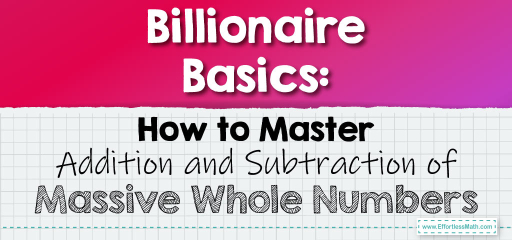# Billionaire Basics: How to Master Addition and Subtraction of Massive Whole Numbers

Handling large numbers can seem daunting, but with a systematic approach, adding and subtracting whole numbers up to the billions can be straightforward. Let's break down the process step by step.## Adding and Subtracting Whole Numbers Up to the Billions

### Example 1:

Add $$3,456,789,012$$ and $$1,234,567,890$$.

Solution Process:

1. Start by aligning the numbers by place value.

2. Add the numbers column by column, starting from the rightmost column (ones place).

3. If the sum in any column is $$10$$ or more, carry over the extra value to the next column on the left.

3,456,789,012

+ 1,234,567,890

_______________

4,691,356,902

The sum is $$4,691,356,902$$.

The Absolute Best Book for 5th Grade Students

### Example 2:

Subtracting Large Numbers

Subtract $$5,678,912,345$$ from $$7,890,123,456$$.

Solution Process:

1. Align the numbers by place value.

2. Subtract column by column, starting from the rightmost column.

3. If the top number in a column is smaller than the bottom number, borrow from the next column on the left.

7,890,123,456

– 5,678,912,345

________________

2,211,211,111

The difference is $$2,211,211,111$$.

Adding and subtracting large numbers, even up to the billions, is a matter of careful alignment and systematic column-by-column operations. With practice, you’ll find that handling these massive numbers becomes second nature. Remember to always align by place value and work from right to left, carrying or borrowing as needed. Keep practicing, and soon you’ll be a pro at managing these billionaire numbers!

### Practice Questions:

1. Add $$4,567,890,123$$ and $$3,456,789,012$$.

2. Subtract $$6,789,012,345$$ from $$8,901,234,567$$.

3. Add $$1,234,567,890$$ and $$9,876,543,210$$.

4. Subtract $$3,456,789,012$$ from $$5,678,901,234$$.

5. Add $$7,890,123,456$$ and $$1,111,111,111$$.

A Perfect Book for Grade 5 Math Word Problems!

1. $$8,024,679,135$$

2. $$2,112,222,222$$

3. $$11,111,111,100$$

4. $$2,222,112,222$$

5. $$9,001,234,567$$

The Best Math Books for Elementary Students

### What people say about "Billionaire Basics: How to Master Addition and Subtraction of Massive Whole Numbers - Effortless Math: We Help Students Learn to LOVE Mathematics"?

No one replied yet.

X
51% OFF

Limited time only!

Save Over 51%

SAVE $15 It was$29.99 now it is \$14.99